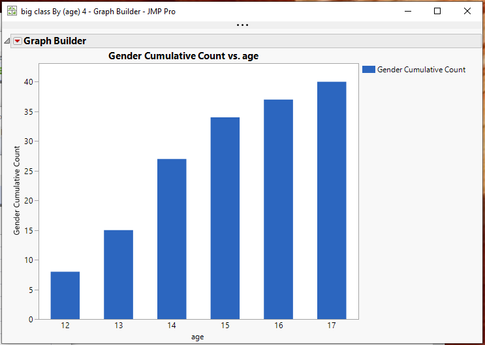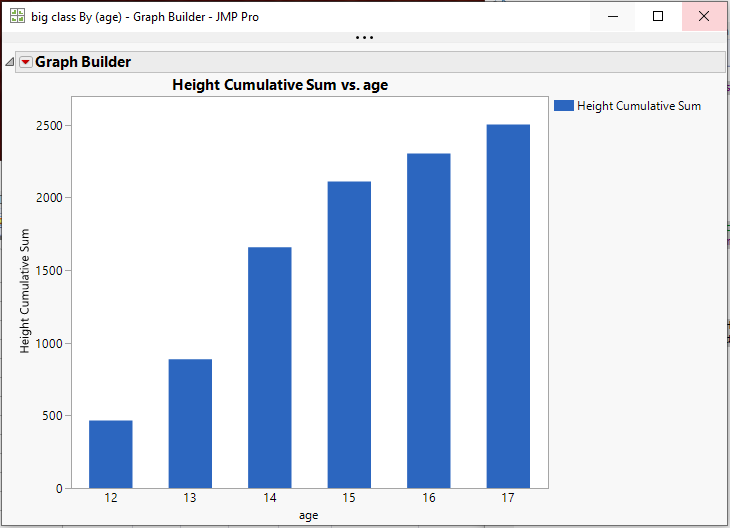Choose Language Hide Translation Bar
Highlighted

## Graph Builder commulative counts of events

How to make simple line graph of commutative occurrences of events month to month? ie month2 = monthe1+month2 etc.

thanks

1 ACCEPTED SOLUTION

Accepted Solutions
Highlighted

## Re: Graph Builder commulative counts of events

Sorry......to do the counts, just change the statistic to N in the Summary()``````names default to here(1);
dt=Open("\$SAMPLE_DATA/big class.jmp");

// Summarize the data
dtSum = dt << Summary(
Group( :age ),
N( :sex ),
Freq( "None" ),
Weight( "None" )
);

// Create the cummulative sum column
dtSum << New Column("Gender Cumulative Count", formula( Col Cumulative Sum( :Name( "N(Sex)" ) )));

// Generate the graph
dtSum << Graph Builder(
Size( 534, 450 ),
Show Control Panel( 0 ),
Variables( X( :age ), Y( :Gender Cumulative Count ) ),
Elements( Bar( X, Y, Legend( 6 ) ) )
);``````

Jim
3 REPLIES 3
Highlighted

## Re: Graph Builder commulative counts of events

Others may have an easier way, but this is how I would do it

1. Use Tables==>Summary to sum the measurement column(s)  for each of the X axis groups.
2. Create a new column in the summary table which is the Col Cumulative Sum() of the summarized measurement column(s)
3. Graph the dataVery easy to do interactively.  But here is the script that produced the above graph

``````names default to here(1);
dt=Open("\$SAMPLE_DATA/big class.jmp");

// Summarize the data
dtSum = dt << Summary(
Group( :age ),
Sum( :height ),
Freq( "None" ),
Weight( "None" )
);

// Create the cummulative sum column
dtSum << New Column("Height Cumulative Sum", formula( Col Cumulative Sum( :Name( "Sum(height)" ) )));

// Generate the graph
dtSum << Graph Builder(
Size( 534, 450 ),
Show Control Panel( 0 ),
Variables( X( :age ), Y( :Height Cumulative Sum ) ),
Elements( Bar( X, Y, Legend( 6 ) ) )
);``````

Jim
Highlighted

## Re: Graph Builder commulative counts of events

Thanks. I knew  how to do it with continuous var. I asked about commutative counts, month1 has 2 events, month2 has 5, month3 has 1, so  I'll show 2-7-8.

Highlighted

## Re: Graph Builder commulative counts of events

Sorry......to do the counts, just change the statistic to N in the Summary()``````names default to here(1);
dt=Open("\$SAMPLE_DATA/big class.jmp");

// Summarize the data
dtSum = dt << Summary(
Group( :age ),
N( :sex ),
Freq( "None" ),
Weight( "None" )
);

// Create the cummulative sum column
dtSum << New Column("Gender Cumulative Count", formula( Col Cumulative Sum( :Name( "N(Sex)" ) )));

// Generate the graph
dtSum << Graph Builder(
Size( 534, 450 ),
Show Control Panel( 0 ),
Variables( X( :age ), Y( :Gender Cumulative Count ) ),
Elements( Bar( X, Y, Legend( 6 ) ) )
);``````

Jim
Article Labels